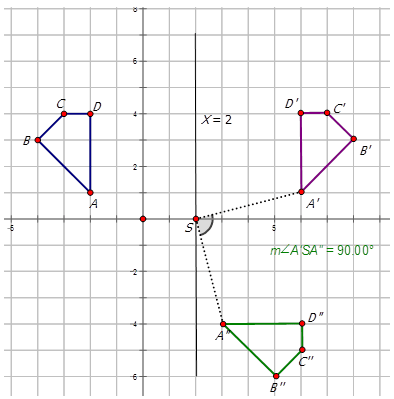# 8.18: Notation for Composite Transformations

•• Contributed by CK12
• CK12

Interpret and use notation for combined transformations

The figure below shows a composite transformation of a trapezoid. Write the mapping rule for the composite transformation.Figure $$\PageIndex{1}$$

In geometry, a transformation is an operation that moves, flips, or changes a shape to create a new shape. A composite transformation is when two or more transformations are performed on a figure (called the preimage) to produce a new figure (called the image). The order of transformations performed in a composite transformation matters.

To describe a composite transformation using notation, state each of the transformations that make up the composite transformation and link them with the symbol $$\circ$$. The transformations are performed in order from right to left. Recall the following notation for translations, reflections, and rotations:

• Translation: $$T_{a,b}: (x,y)\rightarrow (x+a,y+b)$$ is a translation of $$a$$ units to the right and $$b$$ units up.
• Reflection: $$r_{y−axis}(x,y)\rightarrow (−x,y)$$.
• Rotation: $$R_{90^{\circ}}(x,y)=(−y,x)$$

Let's graph the line described below and the composite image defined by $$r_{y−axis} \circ R_{90^{\circ}}$$:

The first translation is a $$90^{\circ}$$ CCW turn about the origin to produce $$X′Y′$$. The second translation is a reflection about the $$y$$-axis to produce $$X′′Y′′$$.Figure $$\PageIndex{2}$$

Now, let's graph the composite images described in the following problems:

1. Image A with vertices $$A(3,5)$$, $$B(4,2)$$ and $$C(1,1)$$ undergoes a composite transformation with mapping rule $$r_{x−axis} \circ r_{y−axis}$$.Figure $$\PageIndex{3}$$
1. Image $$D$$ with vertices $$D(−3,7)$$, $$E(−1,3)$$, $$F(−7,5)$$ and $$G(−5,1)$$ undergoes a composite transformation with mapping rule $$T_{3,4} \circ r_{x−axis}$$.Figure $$\PageIndex{4}$$

Example $$\PageIndex{1}$$

Earlier, you were asked to write the mapping rule for the following composite transformation:Figure $$\PageIndex{5}$$

Solution

The transformation from Image $$A$$ to Image $$B$$ is a reflection across the $$y$$-axis. The notation for this is $$r_{y−axis}$$. The transformation for image B to form image $$C$$ is a rotation about the origin of $$90^{\circ}CW$$. The notation for this transformation is $$R_{270^{\circ}}$$. Therefore, the notation to describe the transformation of Image $$A$$ to Image $$C$$ is $$R_{270^{\circ}} \circ r_{y−axis}$$

Example $$\PageIndex{2}$$

Graph the line $$XY$$ given that $$X(2,−2)$$ and $$Y(3,−4)$$. Also graph the composite image that satisfies the rule $$R_{90^{\circ}} \circ r_{y−axis}$$

Solution

The first transformation is a reflection about the $$y$$-axis to produce $$X′Y′$$. The second transformation is a $$90^{\circ}CCW$$ turn about the origin to produce $$X′′Y′′$$.Figure $$\PageIndex{6}$$

Example $$\PageIndex{3}$$

Describe the composite transformations in the diagram below and write the notation to represent the transformation of figure $$ABCD$$ to $$A′′B′′C′′D′′$$.Figure $$\PageIndex{7}$$

Solution

There are two transformations shown in the diagram. The first transformation is a reflection about the line X=2\) to produce $$A′B′C′D′$$. The second transformation is a $$90^{\circ}$$CW (or $$270^{\circ}CCW$$) rotation about the point $$(2, 0)$$ to produce the figure $$A′′B′′C′′D′′$$. Notation for this composite transformation is:

$$R_{270^{\circ}} \circ r_{x=2}$$

Example $$\PageIndex{4}$$

Describe the composite transformations in the diagram below and write the notation to represent the transformation of figure $$ABC$$ to $$A′′B′′C′′$$.Figure $$\PageIndex{8}$$

Solution

There are two transformations shown in the diagram. The first transformation is a translation of 1 unit to the left and 5 units down to produce $$A′B′C′$$. The second reflection in the $$y$$-axis to produce the figure $$A′′B′′C′′$$. Notation for this composite transformation is:

$$r_{y−axis} \circ T_{−1,−5}$$

## Review

Complete the following table:

Starting Point $$T_{3,−4} \circ R_{90^{\circ}}$$ $$r_{x−axis} \circ r_{y−axis}$$ $$T_{1,6} \circ r_{x-axis}$$ $$r_{y−axis} \circ R_{180^{\circ}}$$
1. $$(1, 4)$$
2. $$(4, 2)$$
3. $$(2, 0)$$
4. $$(-1, 2)$$
5. $$(-2, -3)$$
6. $$(4, -1)$$
7. $$(3, -2)$$
8. $$(5, 4)$$
9. $$(-3, 7)$$
10. $$(0, 0)$$

Write the notation that represents the composite transformation of the preimage $$A$$ to the composite images in the diagrams below.

1.Figure $$\PageIndex{9}$$
2.Figure $$\PageIndex{10}$$
3.Figure $$\PageIndex{11}$$
4.Figure $$\PageIndex{12}$$
5.Figure $$\PageIndex{13}$$